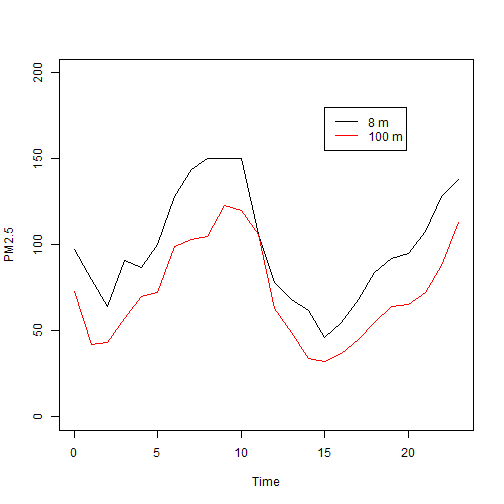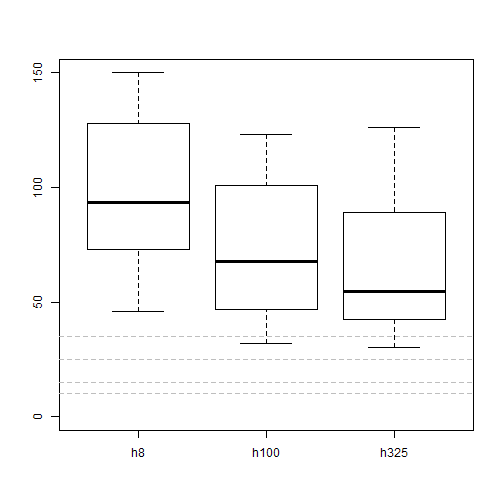``````pm <- read.csv(file = "c:\\R\\data\\dapengde_DummyR_PM25.csv")
plot(x = pm\$time, y = pm\$h8, xlab = "Time", ylab = "PM2.5", type = "l", ylim = c(0,
200))  # 以小时为 x 轴，8 米处的 PM2.5 浓度为 y 轴作图。设定两个坐标的名称。数据点类型为 l 即线型。设定 y 轴范围。
lines(x = pm\$time, y = pm\$h100, col = "red")  # 添加 100 米处 PM2.5 浓度曲线。
legend(x = 15, y = 180, legend = c("8 m", "100 m"), col = c("black", "red"),
lty = 1)  # 添加图例。
````````````练习03.1：请用问号查询 plot、lines、legend 的帮助文件。
``````

``````legend("topleft", legend = c("8 m", "100 m"), col = c("red", "black"), lty = 1) # 图例添加在左上角。
legend(locator(1), legend = c("8 m", "100 m"), col = c("red", "black"), lty = 1) # 用鼠标确定图例的位置。
``````

``````pdf(file = "c:\\R\\data\\output.pdf") # 打开一张pdf的白纸。
plot(x = pm\$time, y = pm\$h8, xlab = "Time", ylab = "PM2.5 at 8 m", type = "l", ylim = c(0, 200)) # 在白纸上画图
dev.off() # 画完了，把纸张收起来
``````

``````pdf(file = "c:\\R\\data\\output2.pdf", width = 8, height = 5) # 设定纸张大小。
plot(x = pm\$time, y = pm\$h8, xlab = "Time", ylab = "PM2.5 at 8 m", type = "l", ylim = c(0, 200), axes=FALSE) # 画图，但坐标轴先空着。
axis(2) # 在左边画出 y 轴。
axis(4) # 在右边画出 y 轴。
axis(1, at = 0 : 23, labels = 0 : 23) # 在下面画出x轴，并在指定位置（at）标出刻度（labels）。
points(x = pm\$time, y = pm\$h100, col = "red", type = "l") # 增加一条线。
points(x = pm\$time, y = pm\$h325, col = "blue", type = "l") # 再增加一条线。
abline(h = c(10, 15 , 25, 35), col = "grey", lty = 2) # 增加几条水平线(世界卫生组织推荐的健康标准值)。
legend("top", legend = c("8 m", "100 m", "325 m"), col = c("red", "black", "blue"), lty = 1) # 添加图例。
box() # 画出边框。
dev.off()
``````

``````boxplot(pm[, c("h8", "h100", "h325")], ylim = c(0, 150))
abline(h = c(10, 15, 25, 35), col = "grey", lty = 2)
````````````练习03.1：请给上面boxplot做的图添加图例，并保存为pdf。
``````

R 的作图功能超级强大，看看这里有很多例子，包含了源代码。

## 有用的信息：

### 32 Responses to “R 菜鸟入门篇 第03篇 画图”

1. 很好狠好 通俗易懂 文字流畅 应该拉你当统计之都的写手

• 欢迎常来，好评是多多益善啊！我是统计学的门外汉一个，这些文字是写给外行看的，只怕会贻笑大方。

2. 写得很流畅，读起来一气呵成，不像有的书，中间参杂了其他的东西，容易断思路。赞一个

• 谢谢。其实是呵了好几口气写成的。

3. pdf(file = “c:\\R\\data\output.pdf”) # 打开一张pdf的白纸。

data后面应该是两个斜杠吧？

• 是的，的确应该是两个斜杠，不知怎么写丢了。谢谢指正！已在原文上修订。

4. 发现一个问题，输出的pdf文件，用福昕阅读器好像看不出颜色的区别，用百度阅读器可以看到

• 这倒是奇怪了，pdf 在不同的阅读器中还有颜色区别？我用 acrobat 看是没有问题的啊。

5. 【求助】
感谢楼主啊，我也是统计的门外汉，在用呢的入门学习R
遇到下面这个问题，请教如何解决？

> pdf(file = “c:\\R\\data\\output.pdf”)
错误于pdf(file = “c:\\R\\data\\output.pdf”) :
无法打开’c:\R\data\output.pdf’文件

• 可能是你的c盘下没有建立\\R\\data 这个文件夹吧。手动建一个。

6. 哈哈，就是这样的，好白的一个问题啊。。。感谢高人！

• 开始的时候都这样，不客气！

7. 说得好详细，很有用！R的书里没有这么具体的用法。支持大鹏~

• 又来捧场了。这才第二条评论，已经显示出水军迹象了。

8. Great, easy to understand. I should read it earlier

• 茉莉？你怎么会摸到这里来？

9. 才发现这篇博，感觉很有用~~~谢谢博主~~~学习R两个月了，在各种搜集资料中~~~是不是可以下此博文系列的PDF文件呢？

• 原来是没有注册呀~~~刚下到了~~~我是各种菜鸟啊~~~T_T

10. 博主文章中有点小错误：
pdf(file = “c:\R\data\output.pdf”
应该为：
pdf(file = “c:\R\data\output.pdf”

• 阁下说得极是，少了个斜线。谢谢指正！已修改。

11. 学习一下

12. 请问你的美元符号怎样打出来的？我用英文半角打出来它老说”invalid”

• 是不是美元符号后面跟的字符敲错了

13. 为什么在plot（x，y）里面输入type=“1”，R 不执行任务呢，画出来的不是线，而是空心点，我是不是哪里出错了。。。。。

• 是L不是1

14. plot(x=pm\$time,y=pm\$h8,xlab=”time”,ylab=”PM2.5″,type=”1″,ylim = c(0,200))

Error in plot.xy(xy, type, …) : invalid plot type ‘1’

请问这是什么原因，不得其解啊。。。

• 已解决，好白的错误

• 祝贺！每干掉一个小怪兽，体能就升了一次级。

15. R作图功能强大那的链接打不开了呢，大鹏博主能更新下吗？

• 那个站没了，请自己搜索类似站点吧！

16. 偶尔看到老师教程，十分棒的新手教程。最近在做3D散点图，用的是R的rgl包，因为可以旋转角度从不同方向观察，还可以做动态图，但是遇到了一个小问题，默认参数作图，做出的图形坐标轴刻度线朝外，并且很长，感觉不是很美观，想修改下坐标轴刻度线，但是试过了“tck”和“tcl”，似乎都不起作用，是因为rgl包的原因，还是3D作图的原因？在论坛也请教了，没人回应，不知道老师能不能帮忙解答下？十分感谢。

17. 请问练习题的答案在哪里看啊？